Next: SIMULTANEOUS ESTIMATION OF WAVELET Up: DECONVOLUTION WITH A KNOWN Previous: Building a more complex

## A generalization for theoperator

Although I have not yet explored the practical implications of this, the operator, previously defined, can be described as a particular case of a more general operator: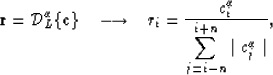(10)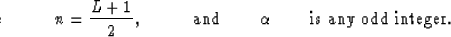The operatorcan be defined as(11)

Figure 7 shows that using this operator with finite values ofis equivalent to the introduction of the damping factor in equation (7). Also we can see that the results obtained with small values ofare similar to those obtained with the predictive deconvolution. An Lp norm deconvolution can be formulated as an iterative, reweighted least-squares inversion with weighting factors given by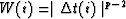, whereare the residuals of the previous iteration (Darche, 1989). Since the value of the exponentwill directly affect the relative weighting of the residuals, we can consider the ICP decon as somehow equivalent to an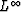norm deconvolution.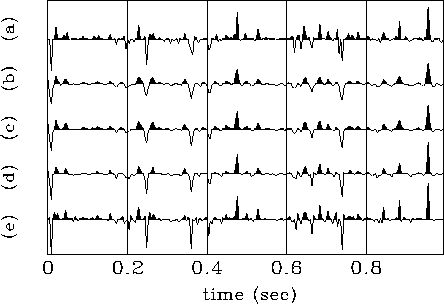knowt3
Figure 7
(a) Original reflectivity series. The other traces correspond to the results of the ICP algorithm using the generalized operator of equation (10) with different values of: (b)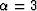,(c), (d)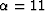,and (e)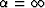.Next: SIMULTANEOUS ESTIMATION OF WAVELET Up: DECONVOLUTION WITH A KNOWN Previous: Building a more complex
Stanford Exploration Project
1/13/1998